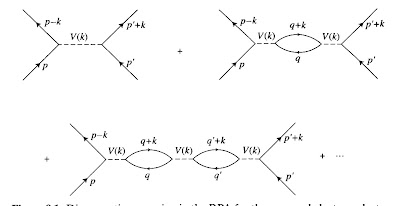## Wednesday, May 19, 2010

### Towards understanding quasi-particle interactions

This weeks reading from Advanced Solid State Physics by Philip Phillips is Chapter 8, Screening and Plasmons.
Some key ideas:

Thomas-Fermi screening gives a first pass explanation of why the independent fermion approximation for the electron gas in a metal works so well. The estimated screening length is shorter than the inter-particle spacing. Consequently, the effective interaction between the quasi-particles is much weaker and shorter range than the large and long-range bare Coulomb interaction.
Key equations are 8.13 and 8.16

Plasma oscillations are collective oscillations of the density. Quanta are plasmons. They arise for a classical plasma (see Ashcroft and Mermin, pp. 19-20). Phillips doesn't make this point clear. Treating the quantum equations of motion for the density at the level of the Random Phase Approximation (RPA) gives their dispersion relation. For elemental metals the plasma frequency corresponds to about 10 eV. For strongly correlated metals such as organic charge transfer salts it can be 1 eV. Metals reflect most light for frequencies less than the plasma frequency (hence they are shiny!).

In two dimensions the screening length and plasma frequency are qualitatively different than from three dimensions.

Linear response theory leads to the fluctuation-dissipation theorem. It is important to realise that that this the quantum generalisation of Einstein's relation between the diffusion constant (fluctuation) and friction (dissipation).
Key equations are 8.38, 8.39, and 8.48

The Lindhardt function (eqn. 8.58) describes the density fluctuations in a non-interacting fermion system.
RPA is equivalent to time-dependent Hartree-Fock.
It leads to the effective interaction U(k,omega) given by 8.71 which has a nice diagrammatic representation shown belowThe frequency dependence of this effective interaction will turn out to be important for understanding superconductivity. In particular it can become attractive at low frequencies because the electron-electron interaction gets screened by the phonons.

Structure functions are the Fourier transform of the density-density correlation functions. They can be measured directly in neutron scattering and so can be compared to experimental data.

More to follow...